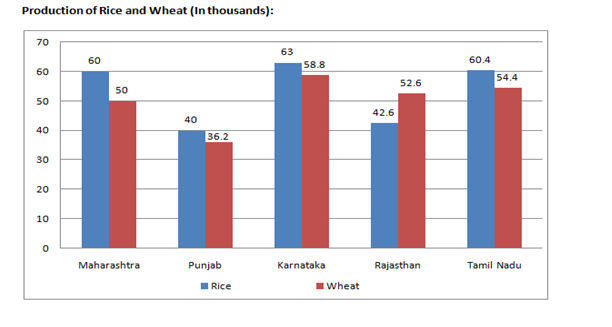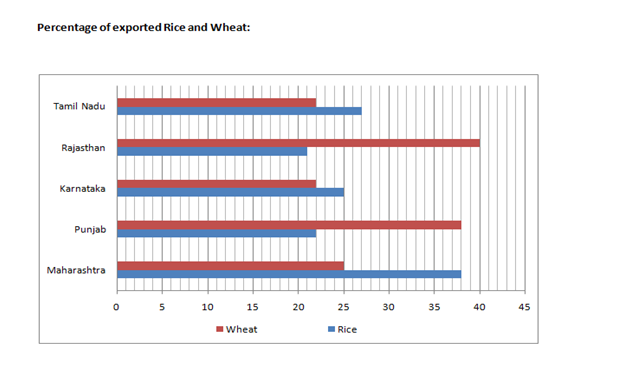# “20-20” Quantitative Aptitude | Crack SBI Clerk 2018 Day-14

Dear Friends, SBI Clerk 2018 Notification has been released we hope you all have started your preparation. Here we have started New Series of Practice Materials specially for SBI Clerk 2018. Aspirants those who are preparing for the exams can use this “20-20” Quantitative Aptitude Questions.

[WpProQuiz 1197]

Click “Start Quiz” to attend these Questions and view Explanation

### Click Here for SBI Clerk Prelims and Mains 2018 – Full Length Mock Test

Direction (Q. 1-5): What will come in the place of question mark (?) in the following number series?

1. 4, 17, 62, ? , 1894, 13267
1. 317
2. 282
3. 128
4. 326
5. None of these
1. 6, 8, ? , 22, 36, 58
1. 16
2. 12
3. 14
4. None of these
5. 13
1. 0.25, 1, 2.25, ? , 6.25, 9, 12.25
1. 5.50
2. 3.50
3. 3.75
4. 4.25
5. None of these
1. 56, 67, 100, ?, 232, 331
1. 135
2. 155
3. 145
4. 127
5. 137
1. 63, 352, 608, ?, 1029, 1198
1. 783
2. 833
3. 939
4. 834
5. 763

Directions(Q. 6 – 10) : What approximate value will come in place of question mark(?) in the given questions ? (Note : you are not expected to calculate the exact value.)

1. 6399 × 1 (5/8) + 353 ÷ ? = 10444
1. 14
2. 22
3. 2
4. 16
5. 8

7).√(624) × 14.02 + √(404) × 15.97 = ?

1. 670
2. 570
3. 710
4. 510
5. 610

8).8461 ÷ 11.99 – 24.01 ÷ (5 / 100) = ?

1. 625
2. 400
3. 250
4. 900
5. 225

9). (14.85% of 679) + (19.9% of 219.83) = ?

1. 115
2. 145
3. 65
4. 105
5. 85

10). 1441÷? + 149.98 × 14.99 = 3006 – 254.91

1. 3
2. 15
3. 25
4. 45
5. 5
1. A certain sum of money amounted to Rs 7200 at 5% in a time in which Rs 4800 amounted to Rs 6336 at rate of 8%. What is the sum in this case considering simple interest rate?
1. Rs 6500
2. Rs 6000
3. Rs 5600
4. Rs 6200
5. None of These
1. In a 100m race, Kranthi beats Shakthi by 10m and Shakthi beats Bhakthi by 5s. What is the speed of Kranthi (in m/s) if it is known that Kranthi runs twice as fast as Bhakthi?
1. 18.0
2. 18.2
3. 17.7
4. 16.2
5. 16.6
1. A and B throw a dice one after the other. If A starts the game, what is the probability that B gets a value more than what A gets?
1. 1/2
2. 6/11
3. 5/12
4. 4/9
5. None of these
1. In z years ago Shashi was one seventh as old as Tinu. In z years from now Tinu will be thrice as old as Shashi. What is the ratio of Tinu’s current age to Shashi’s current age?
1. 4:1
2. 1:4
3. 17:9
4. 8:5
5. Cannot be determined
1. A man purchases 2 horses and 3 cows for Rs 72000. He sells the horses at 15% profit and cows at 5% loss. If in this way he gets a total profit of Rs 8000 then what is the total cost of one horse and one cow?
1. Rs 36667
2. Rs 33667
3. Rs 38774
4. Rs 39774
5. Rs 40664

Direction (Q. 16-20): Study the following graphs carefully and answer the questions that follow:1. What is the average number of Wheat’s produced by Punjab, Karnataka, Rajasthan and Tamil Nadu together?
1. 52500
2. 50500
3. 50100
4. 48600
5. 59800
1. Rice used for Domestic purpose of Maharashtra and Punjab is approximately what percent of the Rice used for domestic purpose of Karnataka and Tamil Nadu?
1. 75 %
2. 63 %
3. 72 %
4. 78 %
5. 80 %
1. What is the average number of Wheat’s exported by Maharashtra, Karnataka and Tamil Nadu?
1. 13568
2. 11298
3. 12468
4. 12048
5. 14158
1. What is the respective ratio between Rice exported by Punjab and Karnataka and Wheat’s exported by same states?
1. 26560 : 28786
2. 20450 : 22357
3. 22810 : 24693
4. 29650 : 31462
5. 24550 : 26692
1. Wheat’s exported by Rajasthan and Tamil Nadu is what per cent more/less than the Wheat’s exported by Maharashtra and Punjab?
1. 20 %
2. 10 %
3. 30%
4. 25%
5. 35 %

Direction (Q. 1-5):

4, 17, 62, ?, 1894, 13267

4 × 3 + 5 = 17

17 × 4 – 6 = 62

62 × 5 + 7 =317

317 × 6 – 8 = 1894

1894 × 7 + 9 = 13267

Hence, 317 will come in the place of question mark.

6, 8, ?, 22, 36, 58

6 + 8 = 14

8 + 14 = 22

14 + 22 = 36

22 + 36 = 58

Hence, 14 will come in the place of question mark.

0.25, 1, 2.25 , ?, 6.25, 9, 12.25

0.5^2= 0.25

1^2 = 1

1.5^2 = 2.25

2^2 = 4

2.5^2 =6.25

3^2 = 9

3.5^2 = 12.25

Hence, 4 will come in the place of question mark.

(Or)

The difference of difference is 0.5

56, 67, 100 , ?, 232, 331

56 + 11 = 67

67 + 33 = 100

100 + 55 = 155

155 + 77 = 232

232 + 99 = 331

Hence, 155 will come in the place of question mark.

63, 352, 608 , ?, 1029, 1198

63 + 289 = 352

352 + 256 = 608

608 + 225 = 833

833 + 196 = 1029

1029 + 169 = 1198

Hence, 833 will come in the place of question mark.

Directions(Q. 6 – 10) :

6399 × (13 / 8) + 353 ÷ ? = 10444

Or, 6400 ×(13 / 8) + (353 / ?) ≈ 10444

Or, 800 × 13 + (353 / ?) ≈ 10444

Or, (353 / ?) = 10444 – 10400 ≈ 44

Or, ? = 353 / 44 = 8.022 ≈ 8

? = √625 ×14 + √400 × 16

≈ 25 × 14 + 20 × 16 = 350 + 320 = 670

? = 8460 ÷ 12 – 24 × (100 / 5)

= 705 – 24 × 20 = 705 – 480 = 225

? = [(15 × 680) / 100] + [(20 × 220) / 100]

= 102 + 44 ≈ 146 ≈ 145

1441 ÷ ? + 149.98 × 14.99

= 3006 – 254.91

Or, 1441 / ? = 2751 – 150 × 15

= 2750 – 2250 = 500

?= 1440 / 500 = 2.88 = 3

Let’s assume that the required time = T years

Hence in 2nd case, (6336 – 4800) = (4800’8’T)/100

=> T = (1536’100)/(4800’8)

=> T = 4 years

Let’s suppose that the principal amount be Rs P in 1St case.

Hence, P + (P'((5’4)/100)) = 7200

=> 1.2P = 7200

=> P = Rs 6000

Let speeds of Kranthi, Shakthi and Bhakthi be denoted by k, s and b respectively.

It is given that Kranthi beats Shakthi by 10m, this means that while Kranthi has travelled 100m, Shakthi has travelled only (100-10) = 90m or 90%.

=> s X t’=0.9 X k X t’

=> s=0.9 X k    …(1)

It is also given that Shakthi beats Bhakthi by 5 secs, this means that:

=> s X t=b X (t+5)=100   …(2)

Also, it is given that: k=2b ..(3)

Using (3) in (1), we get

=> s=0.9 X 2b=1.8b ..(4)

Using (4) in (2), we get

=> 1.8bt=bt+5b =>0.8bt=5b

=> t = 5b/0.8b = 6.25s.

Using this result in (2), we get s = 100/t = 100/6.25 = 16 m/s

Using this result in (1), we get k = sl(0.9) = 16/(0.9) = 17.78 m/s

The favourable cases {(1, 2), (1, 3), (1, 4), (1, 5), (1, 6), (2, 3), (2, 4), (2, 5), (2, 6), (3, 4), (3, 5), (3, 6), (4, 5), (4, 6), (5, 6) }.

Total number of cases = 36

Required probability = 15/36 = 5/12.

Let Shashi and Tinu’s current ages be ‘x’ and ‘y’ years respectively.

Ages z years ago: ‘x – z’ and ‘y – z’

x – z = (1/7) (y – z)

=>7x-7z=y – z

=> 7x – y = 6z  —-(i)

Ages in z years from now: ‘x + z’ and ‘y + z’

3(x + z) = y + z

=> 3x + 3z = y + z

=> y – 3x = 2z

=> 3y – 9x = 6z   …(ii)

Equating (ii) and (i):

=> 7x – y = 3y – 9x

=> 16x = 4y

=> y/x = 4/1

Let the cost price of one horse and one cow be Rs H and Rs C respectively.

According to the question; 2H + 3C = 72000… (1)

According to the question; 2(1.15H) + 3(0.95C) = 80000

=> 2.3H + 2.85C = 80000… (2)

(2) – (1) gives 0.311- 0.15C = 8000

=> H – 0.5C = 80000/3 … (3)

Solving (1) and (3), we get H = 29000 and C = 4667

H + C = 33667

Direction (Q. 16-20):

(36.2 + 58.8 + 52.6 + 54.4)*1000/4

= 202000/4

= 50500

= (60 × 62% + 40 × 78%)/(63 × 75% + 60.4 × 73%) × 100

= (3720 + 3120)/(4725 + 4409) × 100

= 6840 / 9134 × 100

= 74.88%

= 75%

= (50 × 25% + 58.8 × 22%+ 54.4 × 22%)*1000/3

= (12500 + 12936 + 11968)/3

= 37404/3

= 12468

(40 × 22% + 63 × 25 %) : (36.2 × 38% + 58.8 × 22%)

= 24550 : 26692

= (52.6 × 40% + 54.4 × 22%)/(50 × 25% + 36.2 × 38%) × 100

= (2104 + 1196.8)/ (1250 + 1375.6) × 100

= 3300/2625 × 100

= 125.71

= 125%

Required answer = 125 – 100

= 25% more